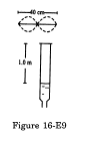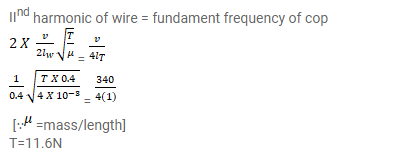# Consider the situation shown in figure.Question:

Consider the situation shown in figure. The wire which has a mass of $4.00 \mathrm{~g}$ oscillates in its second harmonic and sets the air column in the tube into vibrations in its fundamental mode. Assuming that the speed of sound in air is $340 \mathrm{~m} \mathrm{~s}^{-1}$, find the tension in the wire.Solution: